You are currently offline. Some features of the site may not work correctly.

# Sanov's theorem

In information theory, Sanov's theorem gives a bound on the probability of observing an atypical sequence of samples from a given probability… Expand
Wikipedia

## Papers overview

Semantic Scholar uses AI to extract papers important to this topic.
2017
2017
Importance sampling has become an important tool for the computation of extreme quantiles and tail-based risk measures. For… Expand
Is this relevant?
2016
2016
This work is devoted to a vast extension of Sanov's theorem, in Laplace principle form, based on alternatives to the classical… Expand
Is this relevant?
2015
2015
In this paper, we consider the statistics of repeated measurements on the output of a quantum Markov chain. We establish a large… Expand
•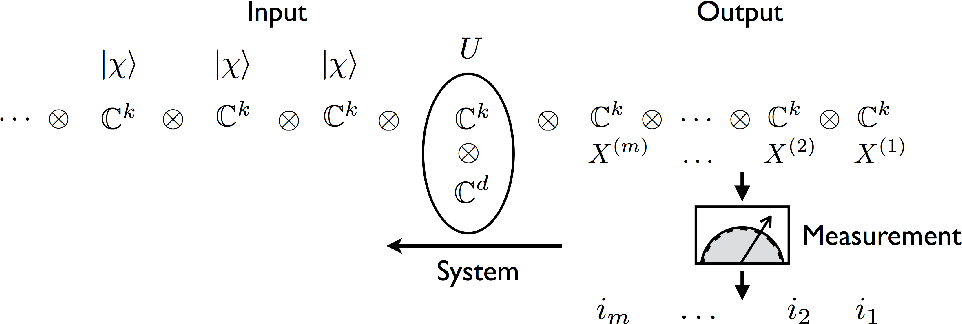•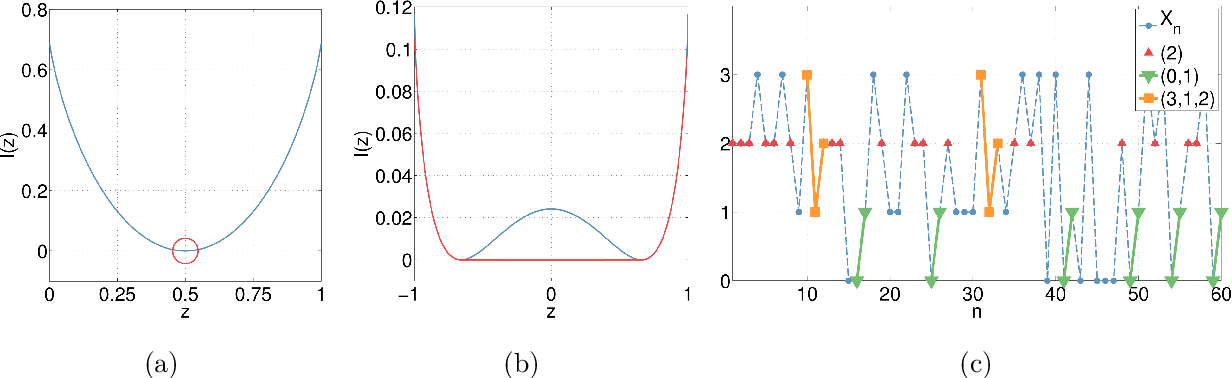•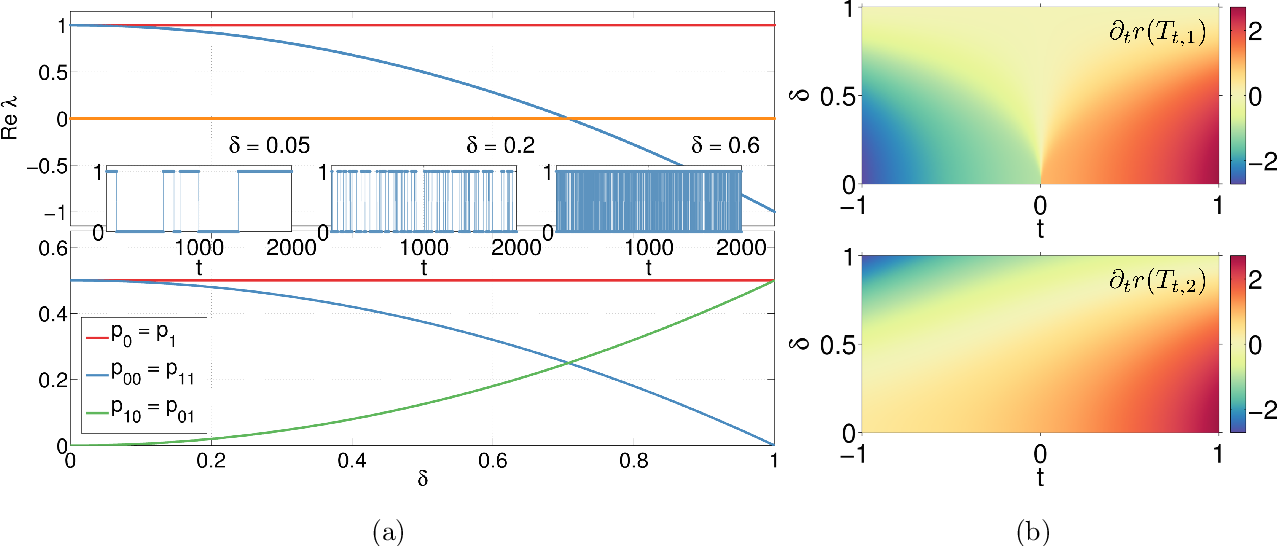•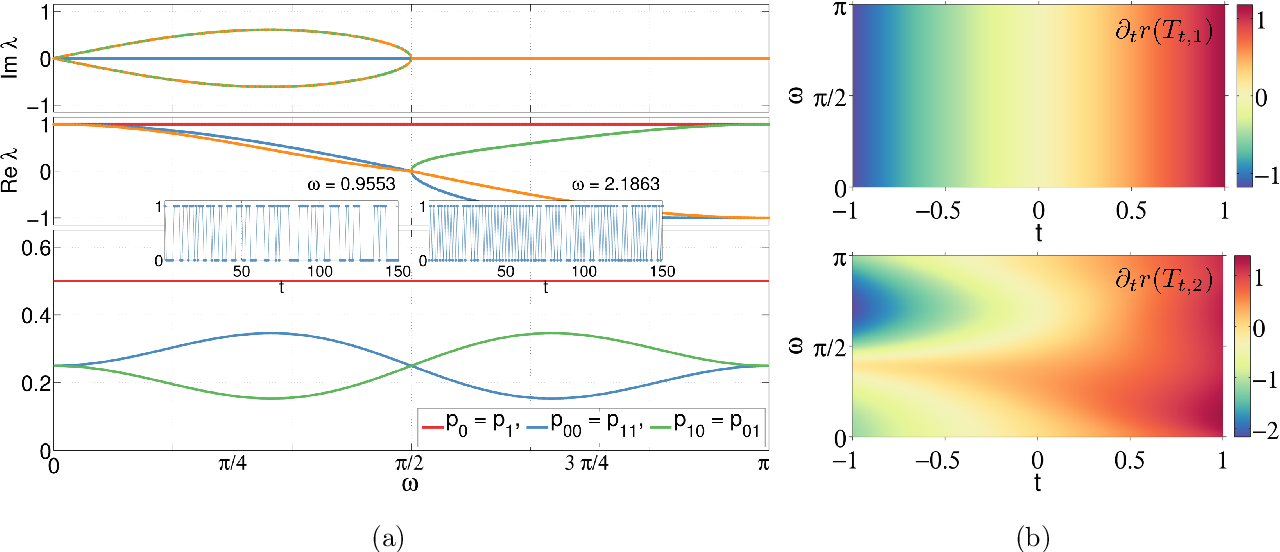Is this relevant?
2014
2014
We report a proof of the quantum Sanov theorem by the elementary application of basic facts about representations of the… Expand
Is this relevant?
2013
2013
We report a proof of the quantum Sanov Theorem by elementary application of basic facts about representations of the symmetric… Expand
Is this relevant?
Highly Cited
2009
Highly Cited
2009
• IEEE/ACM Transactions on Networking
• 2009
We introduce an Internet traffic anomaly detection mechanism based on large deviations results for empirical measures. Using past… Expand
••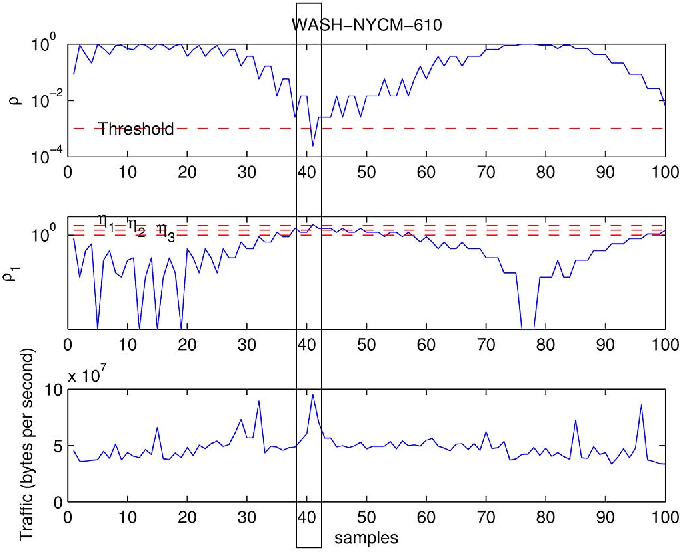•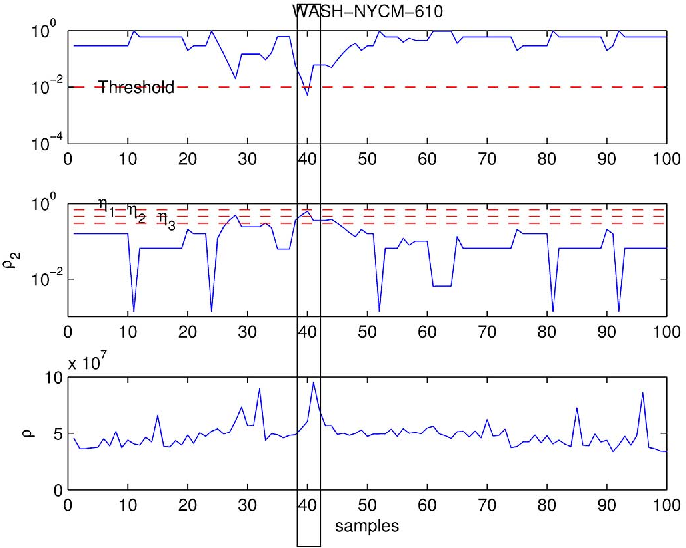•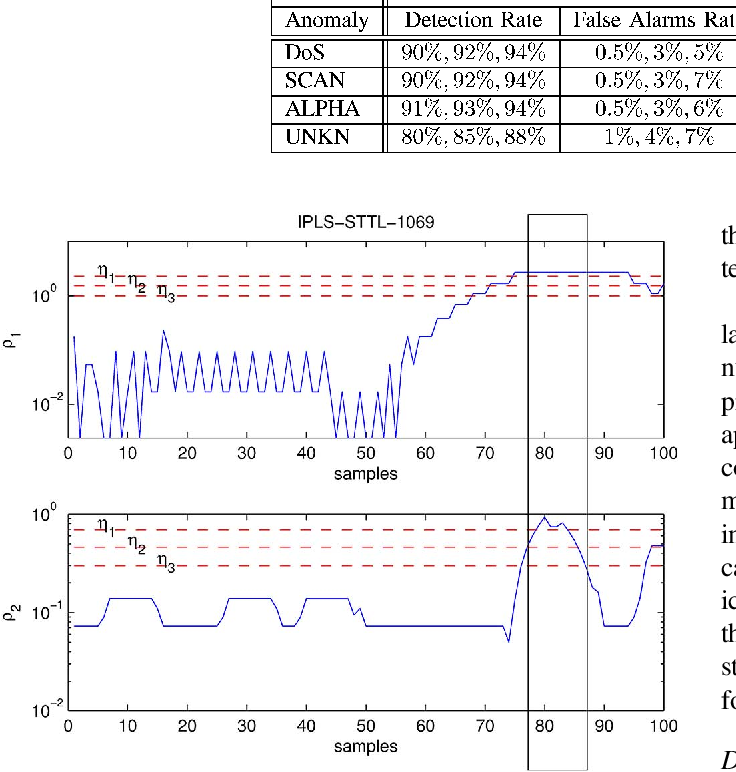•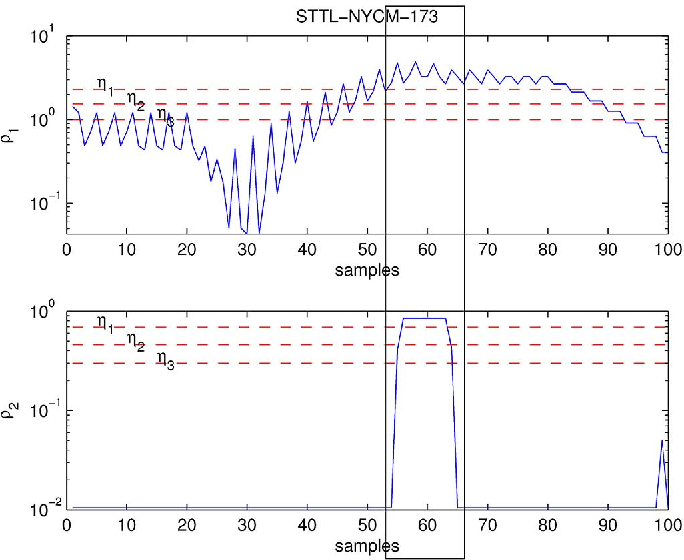Is this relevant?
2009
2009
• IEEE International Symposium on Information…
• 2009
The probability of error in the Koetter-Vardy algebraic soft-decoding algorithm for Reed-Solomon codes is determined by the… Expand
•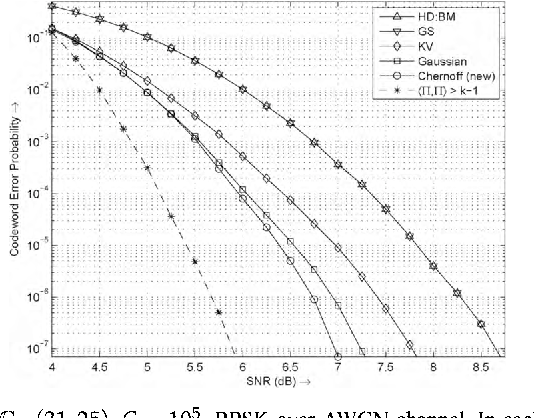•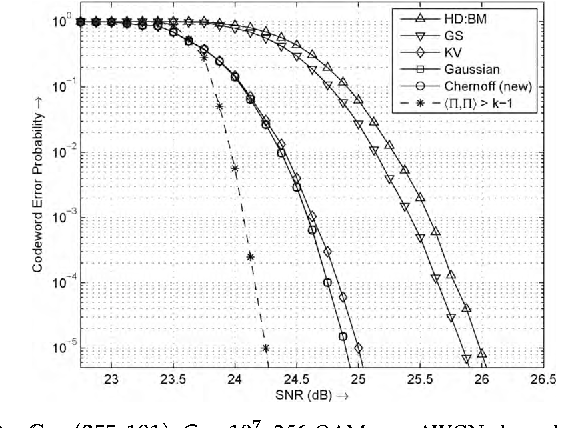Is this relevant?
2003
2003
• IEEE Trans. Information Theory
• 2003
We investigate high-rate quantization for various detection and reconstruction loss criteria. A new distortion measure is… Expand
•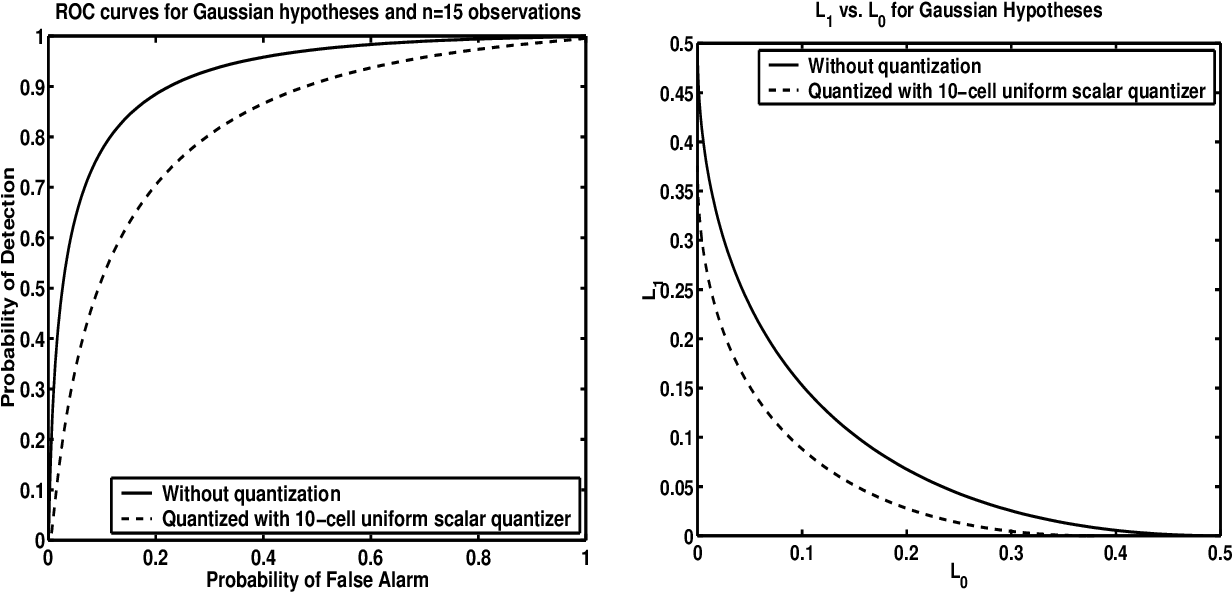•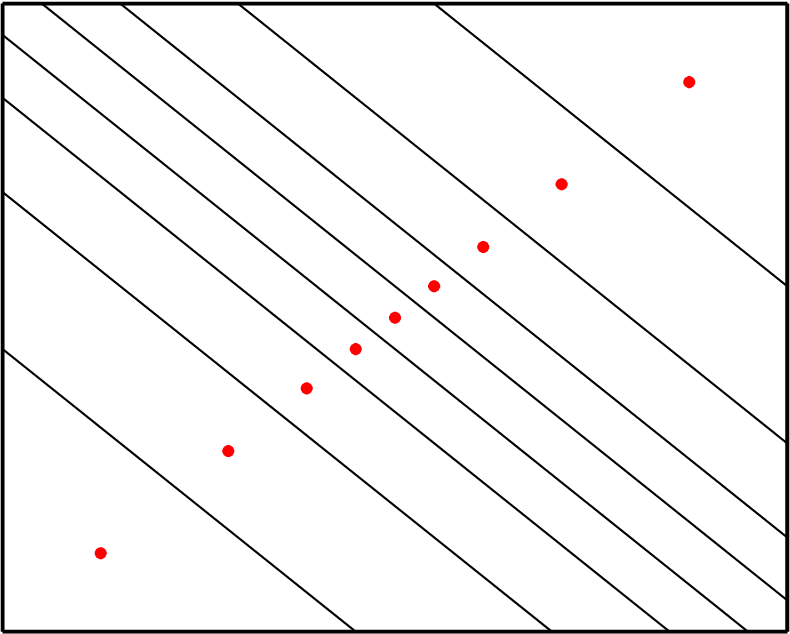•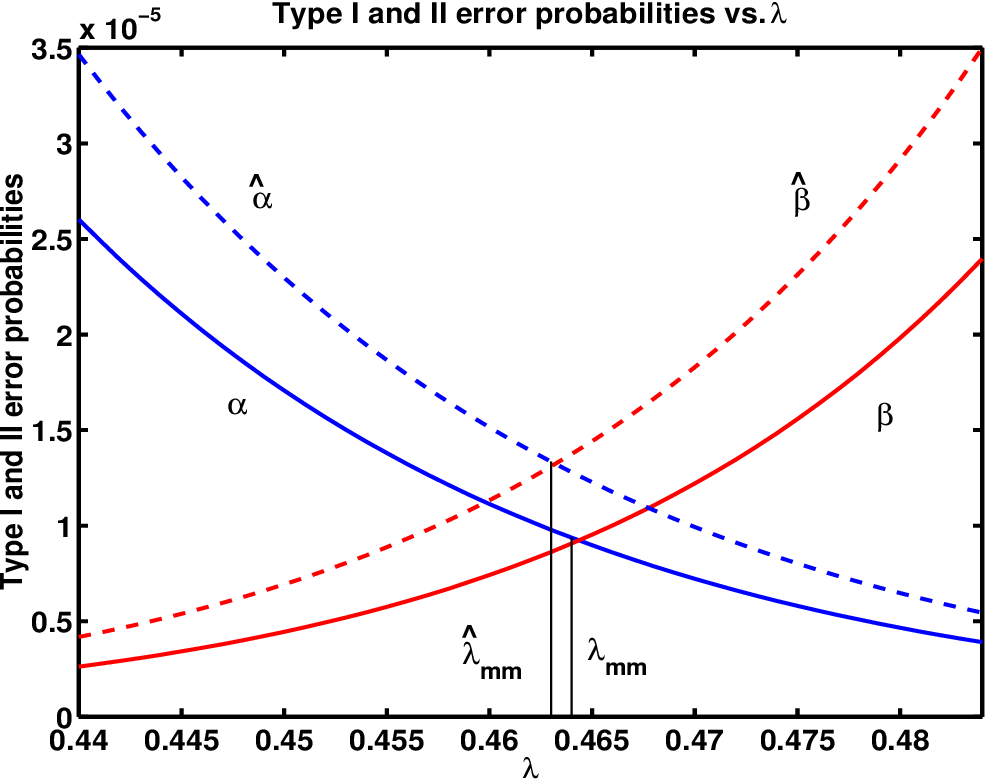•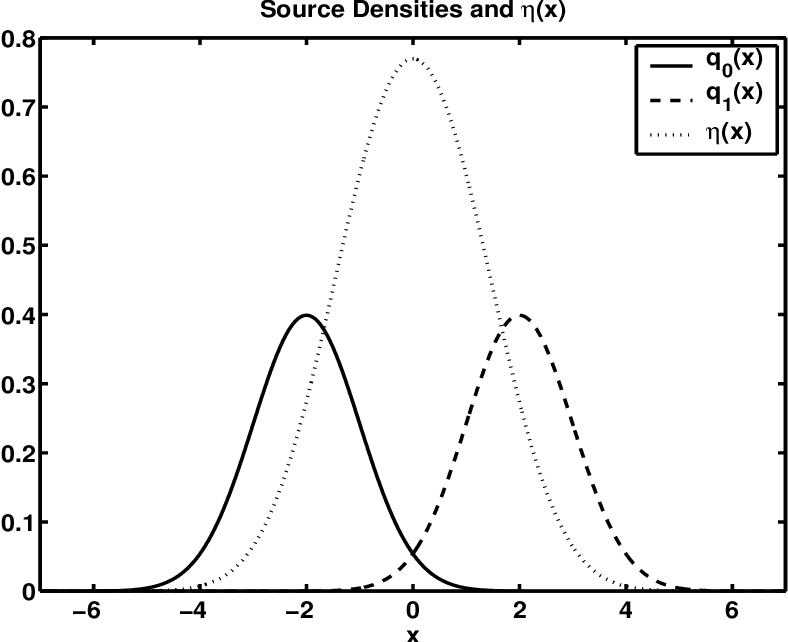•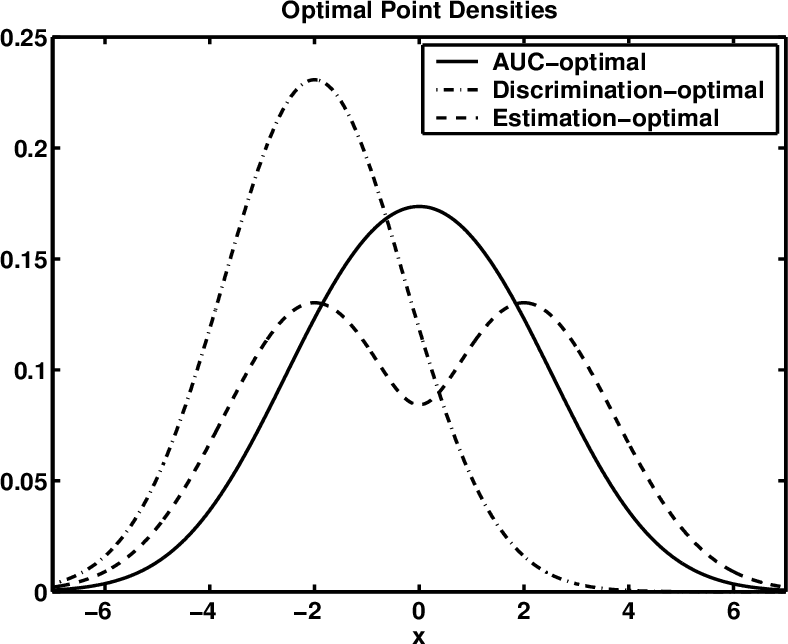Is this relevant?
2000
2000
The physical concept of entropy as it is used in thermodynamics is related to the mathematical formulation of a Shannon entropy… Expand
Is this relevant?
1987
1987
A proof of Shannon's source coding theorem is given using results from large deviation theory. In particular Sanov's theorem on… Expand
Is this relevant?